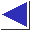# comp.lang.c FAQ list ·Question 3.15

Q: Why does the code

```double degC, degF;
degC = 5 / 9 * (degF - 32);```
keep giving me 0?

A: If both operands of a binary operator are integers, C performs an integer operation, regardless of the type of the rest of the expression. In this case, the integer operation is truncating division, yielding 5 / 9 = 0. (Note, though, that the problem of having subexpressions evaluated in an unexpected type is not restricted to division, nor for that matter to type int.) If you cast one of the operands to float or double, or use a floating-point constant, i.e.

```	degC = (double)5 / 9 * (degF - 32);
or
degC = 5.0 / 9 * (degF - 32);
```
it will work as you expect. Note that the cast must be on one of the operands; casting the result (as in (double)(5 / 9) * (degF - 32)) would not help.